# N-gram language models

## Part 1: Unigram model

• To see the code I wrote for this project, you can check out its Github repo
• For other parts of the project: part 1, part 2, part 3

# Background

Language modeling — that is, predicting the probability of a word in a sentence — is a fundamental task in natural language processing. It is used in many NLP applications such as autocomplete, spelling correction, or text generation.

Currently, language models based on neural networks, especially transformers, are the state of the art: they predict very accurately a word in a sentence based on surrounding words. However, in this project, I will revisit the most classic of language model: the n-gram models.

• In part 1 of the project, I will introduce the unigram model i.e. model based on single words. Then, I will demonstrate the challenge of predicting the probability of “unknown” words — words the model hasn’t been trained on — and the Laplace smoothing method to alleviate this problem. I will then show that this smoothing method can be viewed as model interpolation, which is the combination of different models together.
• In part 2 of the project, I will introduce higher n-gram models and show the effect of model interpolation of these n-gram models.
• In part 3 of the project, aside from interpolating the n-gram models with equal proportions, I will use the expectation-maximization algorithm to find the best weights to combine these models.

# Data

In this project, my training data set — appropriately called train — is “A Game of Thrones”, the first book in the George R. R. Martin fantasy series that inspired the popular TV show of the same name.

Then, I will use two evaluating texts for our language model:

• The first text, called dev1, is the book “A Clash of Kings”, the second book in the same series as the training text. The word dev stands for development, as we will later use these texts to fine-tune our language model.
• The second text, called dev2, is the book “Gone with the Wind”, a 1939 historical fiction book set in the American South, written by Margaret Mitchell. This book is selected to show how our language model will fare when evaluated on a text that is very different from its training text.

# Unigram language model

## What is a unigram?

In natural language processing, an n-gram is a sequence of n words. For example, “statistics” is a unigram (n = 1), “machine learning” is a bigram (n = 2), “natural language processing” is a trigram (n = 3), and so on. For longer n-grams, people just use their lengths to identify them, such as 4-gram, 5-gram, and so on. In this part of the project, we will focus only on language models based on unigrams i.e. single words.

## Training the model

A language model estimates the probability of a word in a sentence, typically based on the the words that have come before it. For example, for the sentence “I have a dream”, our goal is to estimate the probability of each word in the sentence based on the previous words in the same sentence: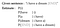For simplicity, all words are lower-cased in the language model, and punctuations are ignored. The [END] token marks the end of the sentence, and will be explained shortly.

The unigram language model makes the following assumptions:

1. The probability of each word is independent of any words before it.
2. Instead, it only depends on the fraction of time this word appears among all the words in the training text. In other words, training the model is nothing but calculating these fractions for all unigrams in the training text.

## Evaluating the model

After estimating all unigram probabilities, we can apply these estimates to calculate the probability of each sentence in the evaluation text: each sentence probability is the product of word probabilities.

We can go further than this and estimate the probability of the entire evaluation text, such as dev1 or dev2. Under the naive assumption that each sentence in the text is independent from other sentences, we can decompose this probability as the product of the sentence probabilities, which in turn are nothing but products of word probabilities.

## The role of ending symbols

As outlined above, our language model not only assigns probabilities to words, but also probabilities to all sentences in a text. As a result, to ensure that the probabilities of all possible sentences sum to 1, we need to add the symbol `[END]` to the end of each sentence and estimate its probability as if it is a real word. This is a rather esoteric detail, and you can read more about its rationale here (page 4).

## Evaluation metric: average log likelihood

When we take the log on both sides of the above equation for probability of the evaluation text, the log probability of the text (also called log likelihood), becomes the sum of the log probabilities for each word. Lastly, we divide this log likelihood by the number of words in the evaluation text to ensure that our metric does not depend on the number of words in the text.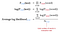For n-gram models, log of base 2 is often used due to its link to information theory (see here, page 21)

As a result, we end up with the metric of average log likelihood, which is simply the average of the trained log probabilities of each word in our evaluation text. In other words, the better our language model is, the probability that it assigns to each word in the evaluation text will be higher on average.

Other common evaluation metrics for language models include cross-entropy and perplexity. However, they still refer to basically the same thing: cross-entropy is the negative of average log likelihood, while perplexity is the exponential of cross-entropy.

# Dealing with unknown unigrams

There is a big problem with the above unigram model: for a unigram that appears in the evaluation text but not in the training text, its count in the training text — hence its probability — will be zero. This will completely implode our unigram model: the log of this zero probability is negative infinity, leading to a negative infinity average log likelihood for the entire model!

## Laplace smoothing

To combat this problem, we will use a simple technique called Laplace smoothing:

1. We add an artificial unigram called [UNK] to the list of unique unigrams in the training text. This represents all the unknown tokens that the model might encounter during evaluation. Of course, the count for this unigram will be zero in the training set, and the unigram vocabulary size — number of unique unigrams — will increase by 1 after this new unigram is added.
2. Next, we add a pseudo-count of k to all the unigrams in our vocabulary. The most common value of k is 1, and this goes by the intuitive name of “add-one smoothing”.

As a result, for each unigram, the numerator of the probability formula will be the raw count of the unigram plus k, the pseudo-count from Laplace smoothing. Furthermore, the denominator will be the total number of words in the training text plus the unigram vocabulary size times k. This is because each unigram in our vocabulary has k added to their counts, which will add a total of (k × vocabulary size) to the total number of unigrams in the training text.

## Effect of Laplace smoothing

Because of the additional pseudo-count k to each unigram, each time the unigram model encounters an unknown word in the evaluation text, it will convert said unigram to the unigram [UNK]. The latter unigram has a count of zero in the training text, but thanks to the pseudo-count k, now has a non-negative probability:

Furthermore, Laplace smoothing also shifts some probabilities from the common tokens to the rare tokens. Imagine two unigrams having counts of 2 and 1, which becomes 3 and 2 respectively after add-one smoothing. The more common unigram previously had double the probability of the less common unigram, but now only has 1.5 times the probability of the other one.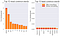Percentages of top 10 most and least common words (before and after add-one smoothing). Left and right bar charts are not plotted at the same scale.

This can be seen from the estimated probabilities of the 10 most common unigrams and the 10 least common unigrams in the training text: after add-one smoothing, the former lose some of their probabilities, while the probabilities of the latter increase significantly relative to their original values. In short, this evens out the probability distribution of unigrams, hence the term “smoothing” in the method’s name.

# Coding the unigram model

## Data pre-processing

Before we apply the unigram model on our texts, we need to split the raw texts (saved as txt files) into individual words. This is often called tokenization, since we are splitting the text into tokens i.e. individual words. The main function to tokenize each text is `tokenize_raw_test`:

• Each line in the text file represents a paragraph. We read each paragraph one at a time, lower its case, and send it to the tokenizer: `generate_tokenized_sentences`.
• Inside the tokenizer, the paragraph is separated into sentences by the `sent_tokenize` function of the NLP library nltk. It can split sentences based on common punctuations, but is also smart enough not to split phrases like “Mr. Smith” into two separate sentences. Nonetheless, it does not fare well with non-standard punctuations like “ or ‘, so we have to replace them with standardized versions that `sent_tokenize` can parse. These replacements are done beforehand by the `replace_characters` function.
• Each sentence is then tokenized into words using a simple `RegexpTokenizer` from nltk. The regex used is `[-\’\w]+` : a valid word contains only alpha-numeric characters (represented by `\w`), dashes (such as snow-covered), and single quotation marks (such as there’s). This unfortunately includes the em-dash, which typically shows up as -- in raw text. As a result, it is transformed into a comma beforehand via the same `replace_characters` function.
• Next, we add a special `[END]` token to the end of each tokenized sentence: `tokenized_sentence.append(‘[END]’)`.
• Lastly, we write each tokenized sentence to the output text file. This tokenized text file is later used to train and evaluate our language models.

Below are the example usages of the pre-processing function, in which each text is tokenized and saved to a new text file:

Here’s the start of training text before tokenization (train_raw.txt):

PROLOGUE
The day was grey and bitter cold, and the dogs would not take the scent.
The big black bitch had taken one sniff at the bear tracks, backed off, and skulked back to the pack with her tail between her legs.

And here it is after tokenization (train_tokenized.txt), in which each tokenized sentence has its own line:

prologue,[END]
the,day,was,grey,and,bitter,cold,and,the,dogs,would,not,take,the,scent,[END]

## Training the model

The formulas for the unigram probabilities are quite simple, but to ensure that they run fast, I have implemented the model as follows:

• First, the `UnigramCounter` class will read each the tokenized training text file one line/sentence at a time. Each unigram in the sentence will be used to increment its count in the `counts` attribute of the class, which is a dict that maps each unigram to its count in the training text. Finally, this class also stores the total number of words in each text via its `token_count` attribute. This class can be used as follows:
• Next, the `UnigramModel` class will take as its input the `UnigramCounter` object associated with the training text. It will then use the unigram counts within the counter’s `counts` dict to calculate the corresponding probabilities of each unigram (based on the Laplace smoothing formula with some specified pseudo-count `k`). The calculated probabilities are stored in the `probs` attribute of the class. All of these calculations can be found in the `train` method of the model, which is used as follows (with `k=1` for add-one smoothing):

## Evaluating the model

Once we have calculated all unigram probabilities, we can apply it to the evaluation texts to calculate an average log likelihood for each text.

• First, we use the same `UnigramCounter` class to count the number of unigrams in each evaluation text.
• The `evaluate` method in the trained `UnigramModel` will be used to calculate the average log likelihood of the model on the evaluation text. It takes in a `UnigramCounter` for the evaluation text. For each unigram in this counter, its count in the evaluation text is multiplied to the log of its probability in the training text (previously stored in the `probs` attribute). If the unigram does not exist in the training vocabulary, we transform it to the [UNK] unigram as outlined earlier.
• For each unigram, we add the above product to the log likelihood of the evaluation text, and repeat this step for all unigrams in the text. The last step is to divide this log likelihood by the number of words in the evaluation text to get the average log likelihood of the text.

The evaluation step for the unigram model on the dev1 and dev2 texts is as follows:

The final result shows that dev1 has an average log likelihood of -9.51, compared to -10.17 for dev2 via the same unigram model.

# Result

## Average log likelihood and similarity of unigram distributions

From the above result, we see that the dev1 text (“A Clash of Kings”) has a higher average log likelihood than dev2 (“Gone with the Wind”) when evaluated by the unigram model trained on “A Game of Thrones” (with add-one smoothing). This makes sense, since it is easier to guess the probability of a word in a text accurately if we already have the probability of that word in a text similar to it.

More formally, we can decompose the average log likelihood formula for the evaluation text as below: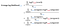Average log likelihood: the average of the trained log probabilities of each word in our evaluation text
• Instead of adding the log probability (estimated from training text) for each word in the evaluation text, we can add them on a unigram basis: each unigram will contribute to the average log likelihood a product of its count in the evaluation text and its probability in the training text. In fact, this is exactly the same method implemented in the `evaluate` method of `UnigramModel`.
• When the denominator of the average log likelihood — the total number of words in the evaluation set — is brought into the summation, it transforms the average log likelihood to nothing but the sum of products between (a) the probability of the unigram in the evaluation text and (b) the log probability of the unigram in the training text.

Written this way, we can see that:

For the average log likelihood to be maximized, the unigram distributions between the training and the evaluation texts have to be as similar as possible.

The simple example below, where the vocabulary consists of only two unigrams — A and B — can demonstrate this principle:

From the example above, we see that:

• A unigram with high training probability (0.9) needs to be coupled with a high evaluation probability (0.7). The log of the training probability will be a small negative number, -0.15, as is their product.
• In contrast, a unigram with low training probability (0.1) should go with a low evaluation probability (0.3). The log of the training probability will be a large negative number, -3.32. However, it is neutralized by the lower evaluation probability of 0.3, and their negative product is minimized.

## Comparison of unigram distributions

When the unigram distribution of the training text (with add-one smoothing) is compared to that of dev1, we see that they have very similar distribution of unigrams, at least for the 100 most common unigrams in the training text:

This is expected, since they are the first and second book from the same fantasy series. A notable exception is that of the unigram ‘ned’, which drops off significantly in dev1. This is no surprise, however, given Ned Stark was executed near the end of the first book.

In contrast, the unigram distribution of dev2 is quite different from the training distribution (see below), since these are two books from very different times, genres, and authors.

Some notable differences among these two distributions:

• The unigram ‘his’ is much less common in dev2, while unigrams like ‘she’ and ‘her’ are much more common. This makes sense, since “Gone with the Wind” mostly revolves around its female protagonist, Scarlett O’Hara.
• There are quite a few unigrams among the 100 most common in the training set, yet have zero probability in dev2. They turn out to all represent the names of Game of Thrones characters, such as ‘jon’, ‘ned’, or ‘sansa’. These characters, of course, never appear in “Gone with the Wind”.

With all these differences, it is no surprise that dev2 has a lower average log likelihood than dev1, since the text used to train the unigram model is much more similar to the latter than the former. This underlines a key principle in choosing dataset to train language models, eloquently stated by Jurafsky & Martin in their NLP book:

Statistical models are likely to be useless as predictors if the training sets and the test sets are as different as Shakespeare and The Wall Street Journal.

# Interpolation of unigram model

Given the noticeable difference in the unigram distributions between train and dev2, can we still improve the simple unigram model in some way? It turns out we can, using the method of model interpolation described below.

## Relationship with Laplace smoothing

Recall the familiar formula of Laplace smoothing, in which each unigram count in the training text is added a pseudo-count of k before its probability is calculated:

This formula can be decomposed and rearranged as follows:

From the re-arranged formula, we can see that the smoothed probability of the unigram is a weighted sum of the un-smoothed unigram probability along with the uniform probability 1/V: the same probability is assigned to all unigrams in the training text, including the unknown unigram [UNK].

In particular, with the training token count of 321468, a unigram vocabulary of 12095, and add-one smoothing (k=1), the Laplace smoothing formula in our case becomes:

In other words, the unigram probability under add-one smoothing is 96.4% of the un-smoothed probability, in addition to a small 3.6% of the uniform probability. As a result, Laplace smoothing can be interpreted as a method of model interpolation: we combine estimates from different models with some corresponding weights to get a final probability estimate.

## Varying interpolation weight

That said, there’s no rule that says we must combine the unigram-uniform models in 96.4–3.6 proportion (as dictated by add-one smoothing). In fact, different combinations of the unigram and uniform models correspond to different pseudo-counts k, as seen in the table below:

From the above table, we see that:

• When k = 0, the original unigram model is left intact. This is equivalent to the un-smoothed unigram model having a weight of 1 in the interpolation.
• As k increases, we ramp up the smoothing of the unigram distribution: more probabilities are taken from the common unigrams to the rare unigrams, leveling out all probabilities. As a result, the combined model becomes less and less like a unigram distribution, and more like a uniform model where all unigrams are assigned the same probability.
• Finally, when the unigram model is completely smoothed, its weight in the interpolation is zero. This is equivalent to adding an infinite pseudo-count to each and every unigram so their probabilities are as equal/uniform as possible.

Now that we understand Laplace smoothing and model interpolation are two sides of the same coin, let’s see if we can apply these methods to improve our unigram model.

# Effects of interpolation

## Reduce overfit of unigram model

As we smooth the unigram model i.e. interpolating it more with the uniform, the model fits less and less well to the training data. This can be seen below for a model with 80–20 unigram-uniform interpolation (orange line). It starts to move away from the un-smoothed unigram model (red line) toward the uniform model (gray line).

However, a benefit of such interpolation is the model becomes less overfit to the training data, and can generalize better to new data. Subjectively, we see that the new model follows the unigram distribution of dev2 (green line) more closely than the original model.

## Reduce variance of estimate

This reduction of overfit can be viewed in a different lens, that of bias-variance trade off (as seen in the familiar graph below):

• On the left, an under-fitting model approximates our data poorly (high bias), but its results remain stable regardless of the data it is trained on (low variance).
• On the right, an over-fitting model approximates our data very well (low bias), but its results can be very different if trained on a different dataset (high variance). As a result, the model that over-fits on the data that it was trained on might give wildly inaccurate result when evaluated on another dataset.

Applying this analogy to our problem, it’s clear that the uniform model is the under-fitting model: it assigns every unigram the same probability, thus ignoring the training data entirely. On the other extreme, the un-smoothed unigram model is the over-fitting model: it gives excellent probability estimates for the unigrams in the training text, but misses the mark for unigrams in a different text.

To visualize the move from one extreme to the other, we can plot the average log-likelihood of our three texts against different interpolations between the uniform and unigram model.

From the accompanying graph, we can see that:

• The pure uniform model (left-hand side of the graph) has very low average log likelihood for all three texts i.e. high bias. However, all three texts have identical average log likelihood from the model. In other words, the variance of the probability estimates is zero, since the uniform model predictably assigns the same probability to all unigrams.
• As more and more of the unigram model is added to the interpolation, the average log likelihood of each text increases in general. However, the average log likelihood between three texts starts to diverge, which indicates an increase in variance.
• Finally, as the interpolated model gets closer to a pure unigram model, the average log likelihood of the training text naturally reaches its maximum. In contrast, the average log likelihood of the evaluation texts (dev1 and dev2) start to steer significantly downward, which further increases the variance in the model’s performance.

For dev1, its average log likelihood reaches the maximum when 91% of the unigram is interpolated with 9% of the uniform. For dev2, the ideal proportion of unigram-uniform model is 81–19. This fits well with our earlier observation that a smoothed unigram model with a similar proportion (80–20) fits better to dev2 than the un-smoothed model does.

In fact, the more different the evaluation text is from the training text, the more we need to interpolate our unigram model with the uniform. This makes sense, since we need to significantly reduce the over-fit of the unigram model so that it can generalize better to a text that is very different from the one it was trained on.

# Final remarks

Doing this project really opens my eyes on how the classical phenomena of machine learning, such as overfit and the bias-variance trade-off, can show up in the field of natural language processing. I hope that you have learn similar lessons after reading my blog post.

In the next few parts of this project, I will extend the unigram model to higher n-gram models (bigram, trigram, and so on), and will show a clever way to interpolate all of these n-gram models together at the end. Please stay tuned!

## References

Jurafsky & Martin’s “Speech and Language Processing” remains the gold standard for a general-purpose NLP textbook, from which I have cited several times in this post. Their chapter on n-gram model is where I got most of my ideas from, and covers much more than my project can hope to do.

A good discussion on model interpolation and its effect on the bias-variance trade-off can be found in this lecture by professor Roni Rosenfeld of Carnegie Mellon University. Note that interpolation of probability estimates is a form of shrinkage, since interpolating an estimate with an estimate of lower variance (such as the uniform) will shrink the variance of the original estimate.

## MTI Technology

A place where MTI-ers can publish ideas about new…

## MTI Technology

A place where MTI-ers can publish ideas about new technologies, agile concepts and their working experiences

Written by

## Khanh Nguyen

Data scientist based in Ho Chi Minh City, Vietnam | dknguyen.com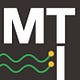## MTI Technology

A place where MTI-ers can publish ideas about new technologies, agile concepts and their working experiences